Wednesday, 29 July 2015

Organic Chemistry- Quiz 1

This quiz for this week is on organic chemistry. You may like to revise the chapter on as well as the chapter on alcohols, carboxylic acids, esters and macromolecules first before attempting the question.

The diagram below shows the structure of macromolecule X.

Which of the following does not react with the monomer of X?
a. hydrogen
b. steam
c. chlorine
d. aqueous sodium hydroxide

Saturday, 25 July 2015

Acids, Bases and Salts - Quiz 1

This quiz for this week is on acids, bases and salts. You may like to revise this chapter first before attempting the question. Click here to revise this chapter.

Which of the following reagents are suitable to be used to prepare a sample of lead (II) sulfate.

a. aqueous lead(II) nitrate and sulfuric acid
b. lead (II) oxide and sulfuric acid
c. lead (II) chloride and aqueous sodium chloride

d. lead (II) oxide and aqueous sodium chloride

Thursday, 23 July 2015

Qualitative Analysis - Testing for cations, anions and gases (Test 1)

The test is on qualitative analysis, testing for cations, anions and gases. To revise this topic, click here. If you would like to try a short quiz on this topic before attempting the test, click here.

The total score for this test is 10 marks. You should complete the test in 10 minutes.

Section A: Multiple Choice (5 marks)
1) Which pair of reagents could be used in a test for chloride ions in aqueous solution?
A. aqueous barium nitrate and nitric acid
B. aqueous barium chloride and hydrochloric acid
C. aqueous silver nitrate and nitric acid
D. aqueous silver nitrate and hydrochloric acid

2) One mole of compound X gives two moles of ions in aqueous solution. X reacts with sodium carbonate to give an acidic gas.

Which of the following could X be?

A. calcium hydroxide
B. hydrochloric acid
C. sodium hydroxide
D. sulfuric acid

3) An aqueous solution of compound Y reacts with aqueous sodium hydroxide to form a blue precipitate. This aqueous solution of compound Y also reacts with acidified barium nitrate solution to give a white precipitate.

What is X?

A. copper(II) chloride
B. copper(II) sulfate
C. iron (II) chloride
D. iron (II) sulfate

4) Which of the following reagent could be used to distinguish aqueous sodium chloride from aqueous sodium nitrate?

A. sulfuric acid
B. acidified barium nitrate solution
C. acidified silver nitrate solution
D. ammonia solution

5) Compound Z is insoluble in water. When nitric acid is added to Z, Z dissolves, giving out an acidic gas. When aqueous ammonia is added to the resultant solution, a white precipitate is formed. This white precipitate then dissolves in excess aqueous ammonia, giving a colourless solution.

What is Z?

B. zinc carbonate
C. lead (II) nitrate
D. zinc nitrate

Section B: Open- ended questions (5 marks)
1) Compound A is a white solid, and dissolves in water to give a colourless solution.

When a solution of A is added to aqueous barium nitrate and nitric acid, a white precipitate is

formed.

When a solution of A is added to aqueous sodium hydroxide dropwise until in excess, a white precipitate is formed. This white precipitate is soluble in excess aqueous sodium hydroxide, giving a colourless solution.

When a solution of A is added to aqueous ammonia dropwise until in excess, a white precipitate is formed. This precipitate is insoluble in excess aqueous ammonia.

i) Predict the identity of A. (2 marks)
ii) Write an chemical equation between a solution of compound A and acidified barium nitrate solution. Include state symbols in your answer. (1 mark)
iii) Write an ionic equation for the formation of the white precipitate, when a solution of compound A is added to aqueous sodium hydroxide.

Sunday, 19 July 2015

Mole Concept - Quiz 1

This question is on mole concept. The full work solution is available here.

Mary would like to find the percentage composition of calcium carbonate in limestone. She added 0.10 g of limestone to 30 cm3 of 0.1 mol/dm3 hydrochloric acid. The resultant solution is then titrated against 0.1 mol/dm3 sodium hydroxide solution. 12 cm3 of sodium hydroxide solution is required to completely neutralize the excess acid. Find the percentage composition of calcium carbonate in the sample of limestone.

Input the answer, without the % sign, corrected to 3 significant figures

Watch the full work solution below:

Friday, 17 July 2015

Qualitative Analysis: Testing for cations, anions and gases - Quiz 1

From this week onwards, we will have a question each week, to test your understanding of the chemistry concepts covered in this blog.

For this week, the topic tested is on Qualitative Analysis - Testing for cations, anions and gases.

You may like to revise this chapter first before attempting the question. Click here to revise this chapter.

When aqueous sodium hyroxide is added to solution X, a white precipitate is formed. This white precipitate dissolves in excess aqueous sodium hydroxide to give a colourless solution. The solution is then heated. No ammonia gas is produced. However, when a piece of aluminium foil is added, ammonia gas is produced.
Which of the following could X be?
a. ammonium nitrate
b. zinc nitrate
c. aluminium chloride
d. lead (II) chloride

Wednesday, 15 July 2015

Rate of reaction

1. Introduction
In order for reaction to take place, reacting particles need to collide in the desired orientation, with a certain minimum of energy This energy is known as activation energy.

Activation energy is the minimum energy required for the reaction to take place.

2. Factors affecting rate of reaction
• concentration of solution
When the concentration of the solution increases, the amount of particles per unit volume increases. Frequency of collision between reacting particles increases. Rate of reaction increases due to increase in frequency of effective collision.
• pressure of gases
When the pressure of gases increases, the amount of particles per unit volume increases. Frequency collision between reacting particles increases. Rate of reaction increases due to increase in frequency of effective collision.
• particle size of solids
When the size of solids decreases, surface area of the solid particles increases, allowing them to collide with other reacting particles more readily. Rate of reaction increases due to increase in frequency of effective collision.
• temperature
When temperature increases, average kinetic energy of the particles increases. Proportion of particles with energy greater than or equal to activation energy increases. Rate of reaction increases due to increase in frequency of effective collision.
• catalyst
Catalyst increases the rate of reaction by providing an alternative pathway, one with a lower activation energy. More particles will have energy greater than or equal to activation energy. Frequency of effective collision increases, and hence rate of reaction increases.

Note that the catalyst remains chemically unchanged at the end of the reaction.

3. Experimental setup to follow rate of reaction
If a reaction gives out gases, the following setup could be used to study the rate of reaction, by obtaining the volume of gas evolved at regular time intervals..

3.1 Measuring change in mass with time, to follow rate of reaction.Rate of reaction experiments - measuring change in mass with time

3.2 Measuring volume of gas produced with time, to follow rate of reaction.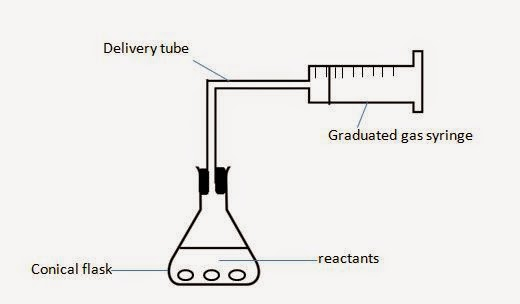Rate of reaction experiments - measuring change in volume of gas with time

4. Interpret data obtained from experiments concerned with speed of reaction
Example. An experiment was set up to study the rate of reaction between excess powdered calcium carbonate and 20 cmof 0.25 mol/dm3 sulfuric acid at 35oC. The volume of carbon dioxide evolved with time is plotted on a graph below:Rate of reaction experiments -  graph of volume of gas evolved with time
i) Explain why does the gradient of the graph decreases until it reaches zero.
The gradient of the graph represents the rate of reaction.
As the reaction progresses, concentration of the acid decreases. Rate of reaction decreases. When all the acid (which is the limiting reagent) is used up, reaction stops, and gradient of graph is zero.

ii) A further experiment, experiment II was repeated, using calcium carbonate granules. Sketch a curve on the graph to show the pattern of results for this experiment. Label the graph II.
Granules are of larger size than powder. The larger the particles size, the smaller the surface area, and the lower the rate of reaction.
There is no change in the amount of calcium carbonate and sulfuric acid used. Hence, maximum volume of gas remains the same.Effect of surface area of solids on rate of reaction

iii) Another experiment, experiment III was repeated, using the same conditions as experiment I, except that 10 cmof 0.5 mol/dm3 of sulfuric acid is used. Sketch a curve on the graph to show the pattern of results for this experiment. Label the graph III.
Concentration of acid increases, hence rate of reaction increases. As sulfuric acid is the limiting reagent, any change in its amount will affect the volume of gas produced.
From the first experiment, the no. of moles of sulfuric acid = 20/1000 x 0.25 = 0.005 mol
For experiment III, the no. of moles of sulfuric acid = 10/1000 x 0.5 = 0.005 mol
Since no. of moles of limiting reagent (sulfuric acid) is the same, volume of gas produced is the same.Effect of concentration on rate of reaction

iv) In experiment IV, the experiment is conducted at 50 oC . Sketch a curve on the graph to show the pattern of results for this experiment. Label the graph IV.
Number of moles of reactant remains the same, only temperature has changed. Hence, no change in maximum volume of gas produced.
The higher the temperature, the higher the rate of reaction. Hence, graph IV will have a higher rate than the first experiment.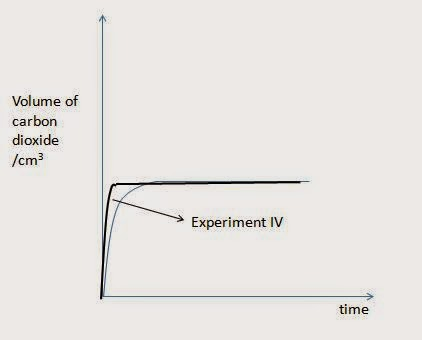Effect of temperature on rate of reaction,

Questions

Particulate theory of matter

1. Solids, liquids and gases
Click here to watch a video from youtube, for an overview on the kinetic particle theory for solids, liquids and gases.

The following is a summary of the particles in solids, liquids and gases:

Solids
• orderly arrangement of particles
• particles are packed closely together, and vibrate about a fixed position. They are also held together by strong forces of attraction
• solid cannot be compressed, and has a fixed shape.
Liquids
• particle are less closely packed together, and are able to move throughout the liquid. Particles are also held together by moderately strong forces of attraction.
• liquids cannot be compressed, and they do not have a fixed shape (take the shape of the container).
Gases
• particles are far apart, and move about randomly and in all directions. Forces of attraction between particles are weak.
• Gases can be compressed (they take the volume of the container), and do not have a fixed shape.
2. Changes in state
Click here to watch a video from youtube, that shows what happens to the particles during state change.

Most substances goes from solid to liquid then to gas as temperature increases, and vice versa when temperature decreases. This means that not many solids sublime on heating. Towards the end of the above video, we see the sublimation of solid carbon dioxide. Carbon dioxide is one of the substances that sublimes (change from solid to gas). Other substances that sublime include iodine and ammonium salts.

During a change in state for pure substances, the temperature remains constant, until all of the substance is changed from one state to another. Take the melting of ice for example. During melting of pure ice, the temperature remains constant, as the heat gained is used to separate the particles in the solid further apart, to form the liquid. It is only when all the pure ice has melted does temperature then start to increase.

3. Diffusion
Diffusion is a process where particles move from a region of higher concentration to a region of lower concentration. Examples of diffusion in our daily life includes being able to smell the perfume when someone applies it some distance away; being able to smell the aroma of coffee some distance away from it.

The rate of diffusion is affected by the density of a substance. The higher the density of a particle, the slower the rate of diffusion. For gases, diffusion is inversely proportional to the molecular mass. The higher the molecular mass, the slower the rate of diffusion. [Hint: This is related to why ammonia diffuses faster than hydrogen chloride].

The rate of diffusion is also affected by temperature. The higher the temperature, the faster the particles move, and as such, the faster the rate of diffusion.

The following is an amazing video on diffusion I found on youtube, which provides what you need to know for secondary school diffusion! Click here to watch the video. The video ends off stating that ammonia diffuses faster than hydrogen chloride. Why is it so?

Ammonia has a formula of NH3. This means that it consists of 1 nitrogen atom and 3 hydrogen atoms per molecule. From the periodic table, the mass number of nitrogen is 14, and the mass number of hydrogen is 1. The molecular mass of ammonia = 14 + 3x 1 = 17.

Hydrogen chloride has a formula of HCl. This means that it consists of 1 chlorine atom and 1 hydrogen atom per molecule. From the periodic table, the mass number of nitrogen is 35.5 and the mass number of hydrogen is 1. The molecular mass of hydrogen chloride = 1 + 35.5 = 36.5.

The lower the molecular mass, the faster the rate of diffusion of the gas. Hence, ammonia diffuses faster than hydrogen chloride.

Questions
- Quizzes and Tests on Particulate Theory of Matter

Air and Atmosphere

1. Composition of Air
The volume composition of gases present in dry air is approximately 78% nitrogen and 21% oxygen. The remainder are noble gases (with argon as the main constituent) and carbon dioxide.

Nitrogen and oxygen can be separated by fractional distillation of liquid air.
Oxygen is often used in the manufacture of steel, and in welding.

2. Common atmospheric pollutants.
Common atmospheric pollutants include carbon monoxide (CO), methane, nitrogen oxides, ozone, sulfur dioxide, and unburnt hydrocarbons.

2.1 Carbon monoxide
Carbon monoxide is produced from incomplete combustion of carbon-containing substances.
Carbon monoxide is odourless and colourless.
It is highly toxic, as it prevents haemoglobin in blood from absorbing.

2.2. Nitrogen oxides
Nitrogen oxides are formed from lightning activity and internal combustion engines.
When dissolved in rainwater, they form acid, which makes rain water acidic (acid rain).

2.3. Sulfur dioxide
Sulfur dioxide is produced from volcanoes and combustion of fossil fuels.
When dissolved in rainwater, sulfur dioxide form acid, which makes rain water acidic (acid rain).

3. Catalytic converters

Catalytic converters are installed in car engines to covert the harmful gases to less harmful ones. These converters consist of catalysts such as platinum and rhodium.
The following are some reactions that take place in the catalytic converter:
Oxides of nitrogen are converted to nitrogen.
Carbon monoxide is converted to carbon dioxide.
Unburnt hydrocarbons are converted to carbon dioxide and steam.
Note that the catalytic converters have no effect on sulfur dioxide.

4. Flue gas desulfurisation
Sulfur dioxide is a gas responsible for the formation of acid rain. Flue gas desulfurisation is a process for removing sulfur dioxide from flue gas. Calcium carbonate reacts with acidic sulfur dioxide to form calcium sulfite (a solid).
calcium carbonate + sulfur dioxide ---> calcium sulfite + carbon dioxide
CaCO3 + SO2 ---> CaSO3 + CO2

5. Chlorofluorocarbons (CFCs) and the ozone layer
The ozone layer prevents excessive ultra violet (UV) light from entering the atmosphere. Excessive UV radiation may result in an increased chance of humans getting skin cancer. CFCs are found to be responsible for depleting the ozone layer.

6. Combustion, respiration and photosynthesis
During combustion, and respiration, oxygen is absorbed while carbon dioxide is released into the atmosphere. During photosynthesis, carbon dioxide is absorbed by plants, and oxygen is liberated.
These processes keep the earth's carbon dioxide level in balance.

7. Greenhouse Gases and Global Warming

Methane and carbon dioxide are greenhouse gases. This means that they trap heat during the night, and keep earth warm. However, when there is excess greenhouse gases, the climate temperature will rise. This is what we call global warming. Global warming could cause the ice caps at the north and south poles to melt, increasing the sea level, and ultimately flooding low lying areas. Some animals may not be suited for the warmer temperatures and may become endangered or extinct.

Questions
- Quizzes and Tests on Air and Atmosphere

Videos
- Causes and Effects of Global Warming

Writing chemical and ionic equations

1. Writing chemical equation
There are generally three steps to writing a chemical equation:
• Determine the reactants and products.
• Write out the formula of the reactants and products.
• Balance the equation.
Note that the first two steps should come naturally, and you should be able to write the equation almost immediately after some practice!

Example. Write a balanced equation for the reaction between calcium carbonate and hydrochloric acid.
Step 1: An acid and carbonate reaction will produce carbon dioxide, water, and salt. In this case, the salt is calcium chloride.

Step 2:
Reactants are calcium carbonate, hydrochloric acid, and their formulae are determined as follows.How to find formula - calcium carbonateHow to find formula  - hydrochloric acid

Products are carbon dioxide, water and calcium chloride.
Formula of carbon dioxide is CO2, and water is H2O.
Formula of calcium chloride is determined as follows:How to find formula - calcium chloride

Step 3: Balance the equation.
calcium carbonate + hydrochloric acid ---> calcium chloride + water + carbon dioxide
CaCO3 + HCl ---> CaCl2 + H2O + CO2
Balancing both sides, you get the final answer:
CaCO3 + 2HCl ---> CaCl2 + H2O + CO2

2. State symbols
To represent the state in a chemical equation, we add in state symbols.
(aq) represents aqueous, which means dissolved in water.;
(l) represents liquid state;
(g) represents gaseous state;
(s) represents solid state.

Let's use the above equation, and add in the state symbols. The following is the balanced chemical equation, which includes state symbol.
CaCO3 (s) + 2HCl (aq) ---> CaCl2 (aq)+ H2O (l)+ CO2(g)

3. Ionic equations
As the name suggest, it is related to ionic compounds. Remember that you only split ionic compounds in the liquid, aqueous or gaseous state into their constituent ions. This is because in the solid states, the ions are held together tightly.

Example. Write the ionic equation for CaCO3 (s) + 2HCl (aq) ---> CaCl2 (aq)+ H2O (l)+ CO2(g).
Step 1: Identify the ionic compounds. They are CaCO3, HCl and CaCl2
Step 2: Identify the ionic compounds that are in liquid, aqueous or gaseous state. They would need to be broken down into the constituent ions.
Only HCl and CaClneeds to be broken down into their constituent ions.
Step 3: Split the liquid, aqueous or gaseous ionic compounds into their constituent ions.
CaCO3 (s) + 2H+ + 2Cl- (aq) ---> Ca2+ (aq) + 2Cl- (aq)+ H2O (l)+ CO2(g)
Step 4: Cancel the same ions that are found on both the reactant and product side.
CaCO3 (s) + 2H+ + 2Cl- (aq) ---> Ca2+ (aq) + 2Cl- (aq)+ H2O (l)+ CO2(g)
Hence, the ionic equation is:

CaCO3 (s) + 2H+ ---> Ca2+ (aq) + H2O (l)+ CO2(g)

Questions
- Quizzes and Test on Writing Chemical and Ionic Equations

Chemical Bonding

1. Ionic compounds
1.1 General
Metals and non- metals form ionic compounds.
Ions are formed when electrons are lost or gained, so that the ion will achieve stable electronic configuration (i.e. 8 valence electrons in the outermost shell, or we call this stable octet configuration. Note that if the outermost shell is the first shell, then 2 electrons in the outermost shell is the stable electronic configuration. We call this stable duplet configuration).
An ionic bond is the electrostatic force of attraction between a positively charged ion (cation) and a negatively charged ion (anion).

1.2 Dot- and- cross diagram of ionic compounds
Next, let's look at how to draw the dot- and- cross diagram for ionic compounds.

Example: Draw the dot- and- cross diagram of magnesium chloride.
Step 1: Determine the type of bonding.
As magnesium is a metal, and chlorine is a non- metal, magnesium chloride is an ionic compound.

Step 2: Determine the charge of the metal and non-metal ion.
Since magnesium is in group II, the charge is +2. Magnesium ion has a formula of Mg2+.
Since chlorine is in group VII, the charge is -1. Chloride ion has a formula of Cl-.

Step 3; Determine the formula of the ionic compound, using the cross method.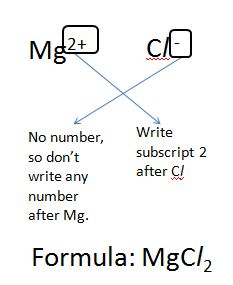How to find formula of ionic compound - magnesium chloride

Step 4: Determine the proton number of the elements.
magnesium has a proton number of 12, and so the electronic configuration of the atom is (2,8,2).
chlorine has a proton number of 17, and so the electronic configuration of the atom is (2,8,7).

Step 5: Determine the electronic configuration of the ions.
Metal will lose the entire last shell of electrons to form ions. Hence, magnesium ion has an electronic configuration of (2,8).
Non- metal will gain electrons until the last shell has 8 electron. Hence, chloride ion has an electronic configuration of (2,8,8).

Step 6: Draw the dot- and- cross diagram!

Dot- and- cross diagram showing all electrons:Structure of magensium chloride - dot- and- cross diagram showing all electrons

Dot- and- cross diagrams showing only outermost electrons:Structure of magnesium chloride - dot- and- cross diagram showing electrons in outermost shells only

1.3 Properties of ionic compounds
• Ionic compounds have high melting and boiling point.
This is due to the strong electrostatic forces of attraction between oppositely charged ions. A large amount of energy is required to overcome these forces during melting or boiling. Thus, ionic compounds have high melting and boiling point.
• Ionic compounds conduct electricity when molten or in aqueous solution, but not in the solid state.
In the solid state, the ions are held together by strong electrostatic attraction and are not free to move about to conduct electricity. When molten or in aqueous solution, the lattice structure of the ionic compound is broken down, and the ions are free to move about to conduct electricity.
• Ionic compounds are generally soluble in water.
(As ionic compounds are able to form ion- dipole interactions with water. --- Note this is not in the syllabus.)

2. Covalent Molecules
2.1 General
A covalent bond is formed by the sharing of electrons between non- metals to achieve state electronic configuration.

There are two types of covalent moelcules. Simple molecules, and giant molecules. In this section, we will be looking at simple molecules.

2.2 Dot- and- cross diagram of simple molecules
Example: Draw the dot- and- cross diagram of water.

Step 1: Determine the type of bonding.
As water and oxygen are both non- metal, the type of bonding present is covalent bonding.

Step 2: Determine the electronic configuration of the atoms.
H has a proton number of 1, thus the electronic configuration is (1). To achieve stable duplet configuration, each H atom requires one more electron. Hence, each H will share 1 electron.
O has a proton number of 8, thus the electronic configuration is (2,6). To achieve stable octet configuration, each O atom requires 2 more electrons. hence, each O will share 2 electrons.

Step 3: Draw the dot- and- cross diagram!

Dot- and- cross diagram showing all electrons:Structure of water - dot- and- cross diagram of water, showing all electrons.

Dot- and- cross diagrams showing only outermost electrons: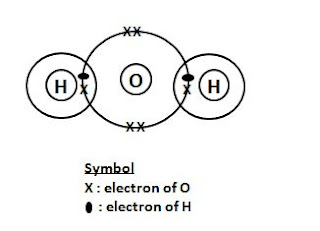structure of water - dot and cross diagram showing outermost electrons only

1.3 Properties of simple covalent molecules

• Simple molecules have low melting and boiling point
This is due to the weak intermolecular forces of attraction. Small amount of energy is required to overcome these forces of attraction. Hence, simple molecules have low melting and boiling point.
[Note: melting and boiling of simple molecules DO NOT involve breaking covalent bonds. The molecules are only separated further apart.]

• Simple molecules do not conduct electricity at all states (solid, liquid and gas).
This is because they do not have free electrons to conduct electricity.
• Simple molecules are generally insoluble in water, but soluble in organic solvents.
3. Metals
The metallic bond consists of a lattice of positive ions in sea of electrons.

Metals can conduct electricity (in all states) due to the presence of mobile electrons.

Metals have high melting and boiling point due to the strong electrostatic forces of attraction between the positive ions and the sea of electrons. Large amount of energy is required to overcome this strong forces of attraction. Hence, metals have high melting and boiling point.

4. Macromolecules or Giant Molecules
These are covalent molecules made up of large number of atoms covalently bonded together. Examples of giant molecules are diamond, graphite, silicon, silicon dioxide and poly(ethene).

4.1. Diamond
In diamond, each carbon atom is covalently bonded to four other carbon atoms, forming a tetrahedral structure. This is then repeated infinitely, forming the giant molecule of diamond. This infinite structure makes diamond hard, and is used as drill bits, and cutting tools.

Diamond cannot conduct electricity as all the valence electrons are used for the covalent bonding, and there is no mobile electron to conduct electricity.

Diamond has a high melting and boiling point, as melting and boiling involves breaking the extensive covalent bonds in diamond. This requires a large amount of energy, resulting in diamond having a high melting and boiling point.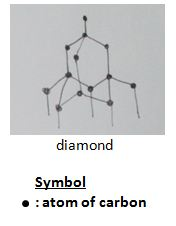Structure of diamond

4.2. Graphite
In graphite, one carbon atom is covalently bonded to three other carbon atoms, forming a hexagonal structure.

Graphite can conduct electricity, as only three out of the four valence electrons in each atom is used for the covalent bonding. The presence of mobile electrons allow graphite to conduct electricity.

Graphite is slippery, as layers of atoms are held together by weak intermolecular forces (van der Waals), and can slide past each other easily. Hence, graphite is used as lubricant.Structure of graphite